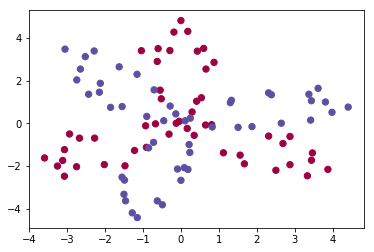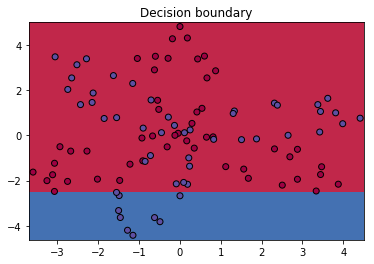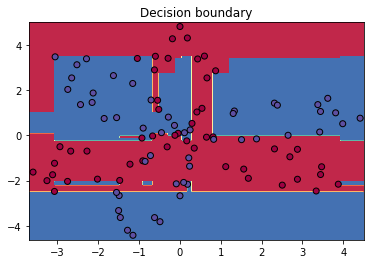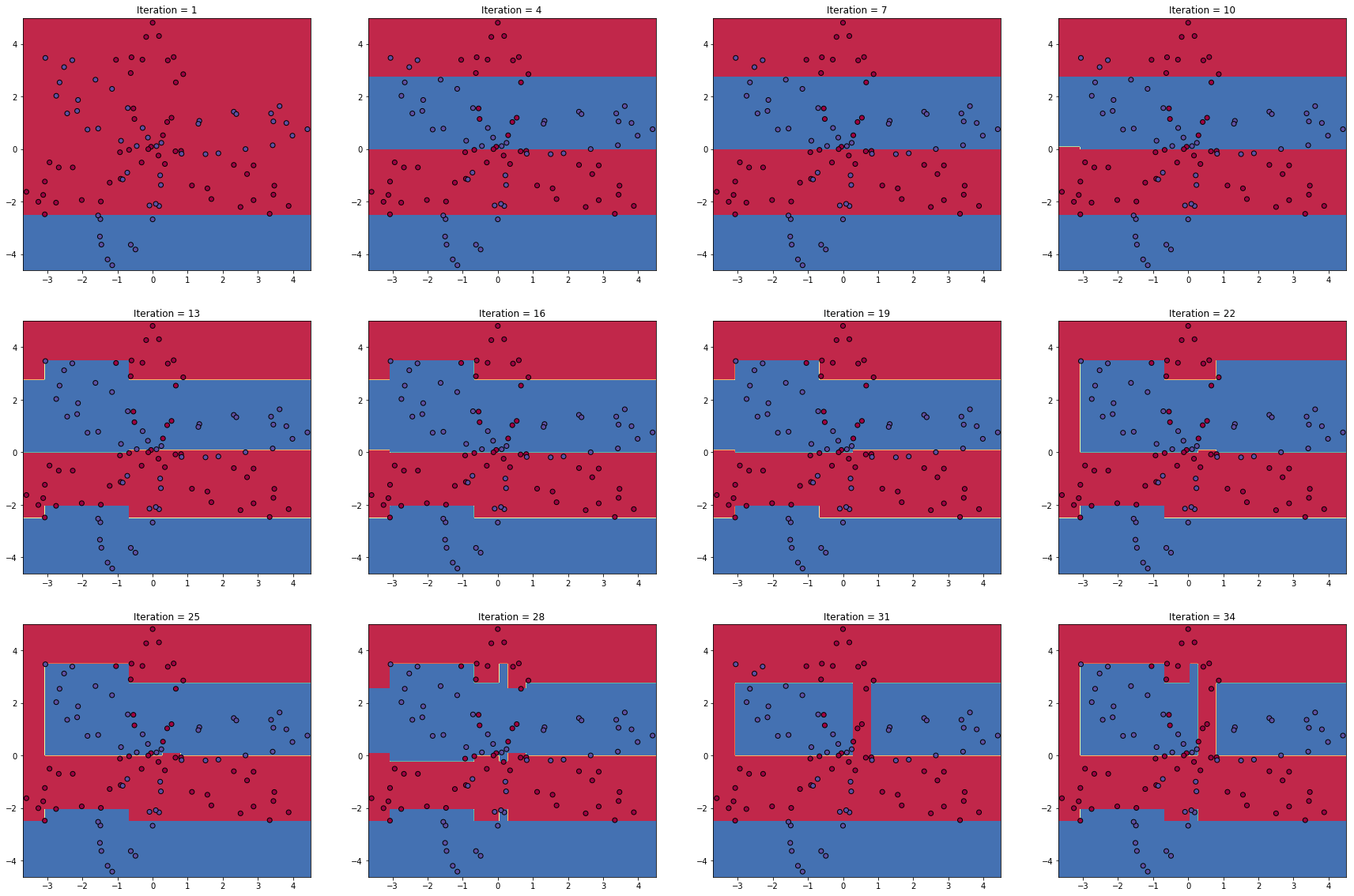### Elan Ding¶

Modified: Aug. 14, 2018 $\newcommand{\bs}{\boldsymbol}$ $\newcommand{\argmin}{\underset{\bs{#1}}{\text{arg min}}\,}$ $\newcommand{\argmax}{\underset{\bs{#1}}{\text{arg max}}\,}$ $\newcommand{\tr}{^{\top}}$ $\newcommand{\norm}{\left|\left|\,#1\,\right|\right|}$ $\newcommand{\given}{\,|\,}$

In this post I will talk about a classification algorithm called AdaBoost, short for Adaptive Boosting. Boosting is one of the most powerful learning techniques. When used with decision trees, it will drastically improve the performance of even a very weak classifier. You will see an example at the end of this post.

Unlike a single classifier, boosting fits the model slowly by using an additive expansion of a set of elementary "basis" functions, in the form of

$$f(\bs{x}) = \sum_{m=1}^M \beta_m b(\bs{x}; \gamma_m) \tag{1}$$

where $\beta_m, m=1,2,..., M$ are real coefficients, and $b(\bs{x}; \gamma)$ is a real valued smooth function that usually have a simple form, such as linear, characterized by the parameter $\gamma$. Perhaps the best example is a single-layered neural network, as described in my previous post, where the function $b(\bs{x}; \gamma)$ is

$$\sigma(\bs{W}^{} \bs{a}^{} + \bs{b}^{}) = b(\gamma_0 + \gamma_1\tr \bs{x})$$

where $\sigma$ is the sigmoud function, $\gamma = (\gamma_0, \gamma_1)'$ reprenets both the weight $\bs{W}^{}$ and the bias $\bs{b}^{}$, and $\bs{x}$ here is the first hidden activation nodes $\bs{a}^{}$.

Similar to the neural networks, the parameters are found by minimizing the total loss

$$\sum_{i=1}^N L\left(y^{(i)}, f(\bs{x}^{(i)})\right) = \sum_{i=1}^N L\left(y^{(i)}, \sum_{m=1}^M \beta_m b(\bs{x}; \gamma_m)\right). \tag{2}$$

where $L$ can be either the RSS or the cross-entropy. (Recall in a neural network, we used the the cross entropy between the empirical distribution and a binomial distribution.) As we have seen earlier, finding these parameters via back propagation is computationally expensive (via gradient descent), and to make the matters even worse, sometimes the loss cannot be differentiated, such as when $b(\bs{x}; \gamma)$ represents a decision tree. To solve this issue we use a new powerful method called the forward stagewise additive modeling.

Algorithm 1 Forward Stagewise Additive Modeling

1. Initialize $f_0(\bs{x}) = 0$
2. For $m=1$ to $M$:

(a) Compute $$(\beta_m, \gamma_m) = \argmin{\beta, \gamma} \sum_{i=1}^N L(y^{(i)}, f_{m-1} (\bs{x}^{(i)}) + \beta b(\bs{x}^{(i)}; \gamma))$$ (b) Update the predition

$$f_m(\bs{x}) = f_{m-1}(\bs{x}) + \beta_m b(\bs{x}; \gamma_m).$$

What is happening here? At the first iteration, we look for coefficients $\beta_1$ and $\gamma_1$ that minimizes the loss

$$\sum_{i=1}^N L(y^{(i)}, \beta b(\bs{x}^{(i)}; \gamma)).$$

This is similar to fitting a model to the original dataset, and we get the first non-trivial model $f_1(\bs{x})$. Since $b(\bs{x}, \gamma)$ is a weak model, the first prediction function $f_1$ is not very accurate. During the second iteration, we find $\beta_2$ and $\gamma_2$ that minimizes the loss

$$\sum_{i=1}^N L(y^{(i)}, f_1(\bs{x}^{(i)}) + \beta b(\bs{x}^{(i)}; \gamma)) = \sum_{i=1}^N L(r^{(i)}_1, \beta b(\bs{x}^{(i)}; \gamma)),$$ where $r_1^{(i)} = y^{(i)} - f_1(\bs{x}^{(i)})$ is the residual of the first predictor $f_1$. In general, during the $m$th iteration, we simply need to find

$$(\beta_m, \gamma_m) = \argmin{\beta, \gamma} \sum_{i=1}^N L(r_{m-1}^{(i)}, \beta b(\bs{x}^{(i)}; \gamma)). \tag{3}$$

where $r_{m-1}^{(i)} = y^{(i)} - f_{m-1}(\bs{x}^{(i)})$ is the residual of $f_{m-1}$.

What an ingenious idea! Whichever portions of the output that $f_1$ failed to predict are assigned to $f_2$. Likewise, the second residual, which captures the parts of the output that $f_2$ failed to predict are fitted with $f_3$, and so on.

## Exponential Loss¶

If you have read Chapter 10 from ESLII, then you have probably come across equation (10.18) and feel perplexed. Indeed, ESLII is a very dense book and some pages require a lot of re-reading to fully understand them. Here I will help you better understand the material by offering my own interpretation.

Having reduced our problem to the form in (3), we can now deal with the loss function. It turns out that using the usual RSS for the loss is generally not a good idea for classification. Instead, we turn to the exponential loss,

$$L(y, f(\bs{x})) = \text{exp}(-yf(\bs{x})), \tag{4}$$

where the output $Y \in \{-1, 1\}$ is a binary random variable. Indeed this is a valid loss function. When $y=1$, and $f(\bs{x})$ have the same sign as $y$, the loss is smaller compared to when $f(\bs{x})$ and $y$ have different signs. Furthermore, note that the expected value of the exponential loss is

$$E_{Y\given \bs{x}} \text{exp}(-Yf(\bs{x})) = \text{exp}(-f(\bs{x})) P(Y=1\given \bs{x}) + \text{exp}(f(\bs{x})) P(Y=-1\given \bs{x})$$

Taking the derivative with respect to $f(\bs{x})$, set it equal to 0, and solve for $f$, we obtain

$$f^*(\bs{x}) = \argmin{f(\bs{x})} E_{Y\given \bs{x}} \text{exp}(-Yf(\bs{x})) = \frac{1}{2} \log \frac{P(Y=1 \given \bs{x})}{P(Y=-1\given \bs{x})} \tag{5}$$

We see that the expected exponential loss is minimized by $f^*(x)$, the logit transformation of $p(Y\given \bs{x})$. Hence, we can simply make predictions of $Y$ based on the sign of $f^*(\bs{x})$. Rewriting this, we get

$$p(\bs{x}) = P(Y=1 \given \bs{x}) = \frac{1}{1+\text{exp}(-2f^*(\bs{x}))}.\tag{6}$$

This is the population's true distribution of $Y$, which is similar to binomail (except that the output is $\{-1, 1\}$) with success probability $p(\bs{x})$. Inspired by this form, we let our modeled distribution to have the same distribution with success probability given by

$$p_{\text{model}}(\bs{x}; f) = \frac{1}{1+\text{exp}(-2 f(\bs{x}))}.\tag{7}$$

In fact, when $f(\bs{x})$ is a linear function, this is exactly like a logistic regression. Next, to make the distribution truly binomial, we let $Y' = (Y+1)/2 \in \{0, 1\}$. Given a training example $(\bs{x}, y)$, the empirical distribution is simply $\widehat{p}(y)=1$, so the binomial cross-entropy is given by

\begin{aligned} H(\widehat{p}, p_{\text{model}}) &= E_{y'\sim \widehat{p}} -\log(p_{\text{model}}(y'))\\ & = -\log p_{\text{model}}(\bs{x})^{y'}(1-p_{\text{model}}(\bs{x}))^{1-y'} \\ & = - y'\log p_{\text{model}}(\bs{x}) - (1-y')\log(1-p_{\text{model}}(\bs{x})) \\ & = \log\left(1 + \text{exp}(-2yf(\bs{x}))\right), \end{aligned} \tag{8}

where the last equality follows by noting that if $y=1$, then $y'=1$, and the cross entropy is

$$-\log p_{\text{model}}(\bs{x}) = \log\left(1+\text{exp}(-2f(\bs{x}))\right).$$

Similarly, if $y=-1$, then $y'=0$, and the cross entropy is

$$-\log(1-p_{\text{model}}(\bs{x})) = -\log \frac{\text{exp}(-2f(\bs{x}))}{1+\text{exp}(-2f(\bs{x}))} = \log\left(1+\text{exp}(2f(\bs{x}))\right).$$

Let's reflect on what we have so far. From (5), we see that the expected exponential loss is minimized by $f^*$. Using the binomial cross entropy, we arrived at (8), whose minimum also occurs at $f^*$ since by comparing $(6)$ and $(7)$, we see that $p_{\text{model}}$ is maximized precisely when $f = f^*$.

Therefore, in the population level, we see that the loss minimization using the exponential loss is equivalent to using the binomial cross-entropy. The only difference is that now the binary variable $Y$ takes values $\{-1, 1\}$ instead of $\{0, 1\}$.

The main attraction of using the exponential loss is computational. In AdaBoost, the individual weak classifiers $b_m(\bs{x}; \gamma)$ is rewritten as $G_m(\bs{x}) \in \{-1, 1\}$. Using the exponential loss function, we solve

\begin{aligned} (\beta_m, G_m) &= \argmin{\beta, G} \sum_{i=1}^N \text{exp} \left[-y^{(i)}(f_{m-1}(\bs{x}^{(i)}) + \beta G(\bs{x}^{(i)})) \right] \\ &= \argmin{\beta, G} \sum_{i=1}^N w_m^{(i)} \text{exp} \left(-\beta y^{(i)} G(\bs{x}^{(i)}) \right) \end{aligned}\tag{9} where $w_m^{(i)} = \text{exp}(-y^{(i)}f_{m-1}(\bs{x}^{(i)}))$ are constants that does not depend on $\beta$ nor $G$.

Since $y$ and $G(\bs{x})$ can be either $1$ or $-1$, after fixing $\beta>0$, we can rewrite the summation in $(9)$ as

$$e^{-\beta} \sum_{y^{(i)} = G(\bs{x}^{(i)})} w_m^{(i)} + e^{\beta} \sum_{y^{(i)} \neq G(\bs{x}^{(i)})} w_m^{(i)} = (e^{\beta} - e^{-\beta}) \sum_{i=1}^N w_m^{(i)} I(y^{(i)} \neq G(\bs{x}^{(i)})) + e^{-\beta} \sum_{i=1}^N w_m^{(i)}.\tag{10}$$

Hence when $\beta>0$ is fixed, to minimize (10), we have

$$G_m = \argmin{G} \sum_{i=1}^N w_m^{(i)} I(y^{(i)} \neq G(\bs{x}^{(i)})). \tag{11}$$

Now differentiate (10) with respect to $\beta$, set it equal to 0, and solve for $\beta$. We get:

$$\beta_m = \frac{1}{2} \log \frac{1 - \text{err}_m}{\text{err}_m} \tag{12}$$

where $\text{err}_m$ is the minimized weighted error rate

$$\text{err}_m = \frac{\sum_{i=1}^N w_m^{(i)} I(y^{(i)} \neq G_m(\bs{x}^{(i)}))}{\sum_{i=1}^N w_m^{(i)}},\tag{12}$$

and $G_m$ is given by equation (11).

After obtaining both $\beta_m$ and $G_m$, we update our approximation according to Algorithm 1 by

$$f_m(\bs{x}) = f_{m-1}(\bs{x}) + \beta_m G_m(\bs{x}).$$

At the same time, we update the weights by

$$w_{m+1}^{(i)} = w_{m}^{(i)} \text{exp}(-\beta_m y^{(i)} G_m(\bs{x}^{(i)})). \tag{13}$$

Using a clever trick by re-writing $$-y^{(i)} G_m(\bs{x}^{(i)}) = 2 I(y^{(i)} \neq G_m(\bs{x}^{(i)}))-1,$$

we can rewrite (13) as

$$w_{m+1}^{(i)} = w_m^{(i)}\, \text{exp} (2\beta_m I(y^{(i)} \neq G_m(\bs{x}^{(i)}))\,\text{exp}(-\beta_m).$$

Note that the term $\text{exp}(-\beta_m)$ is multiplied to all weights, so it has no effect. Setting $\alpha_m = 2\beta_m$, we have finally arrived at one of the most popular boosted algorithm, the AdaBoost.

1. Initialize the weights for the training set $w^{(i)} = 1/N, i=1,..., N$.
2. For $m=1$ to $M$:

(a) Fit a classifier $G_m(\bs{x})$ to the training set using the weights $w^{(i)}$

(b) Compute the weighted error term $$\text{err}_m = \frac{\sum_{i=1}^N w^{(i)} I(y^{(i)} \neq G_m(\bs{x}^{(i)}))}{\sum_{i=1}^N w^{(i)}}.$$

(c) Compute log odds of the error $$\alpha_m = \log \frac{1-\text{err}_m}{\text{err}_m}.$$

(d) Update the weights: For $i=1$ to $N$: $$w^{(i)} := w^{(i)}\, \text{exp}(\alpha_m I(y^{(i)} \neq G_m(\bs{x}^{(i)}))).$$

1. Output the prediction using the sign of the weighted expansion: $$G(\bs{x}) = \text{sign}\left(\sum_{m=1}^M \alpha_m G_m(\bs{x}) \right).$$

## Implementation¶

Let's do a quick implementation to show the power of AdaBoost! Let's generate the same data set as in the previous post. The only difference this time is that the class labels are in $\{-1, 1\}$ instead of $\{0, 1\}$. This matters for AdaBoost!

In :
import numpy as np
import matplotlib.pyplot as plt
%matplotlib inline

In :
def load_data():
np.random.seed(1)
m = 100 # number of examples
N = int(m/2) # number of points per class
X = np.zeros((m,2)) # data matrix where each row is a single example
Y = np.zeros(m) # labels vector (-1 for red, 1 for blue)

for j in range(2):
ix = range(N*j,N*(j+1))
t = np.linspace(0, 2*3.12, N) # theta
r = 4*np.cos(3*(t+j*3.14/4)) + np.random.randn(N)*0.5 # radius
X[ix] = np.c_[r*np.sin(t), r*np.cos(t)]
Y[ix] = 2*j-1

return X, Y

In :
# load and plot data
plt.scatter(X[:,0], X[:,1], c=y, s=40, cmap=plt.cm.Spectral)

Out:
<matplotlib.collections.PathCollection at 0x7f3143fb8cc0>Next, we are going to fit a "weak" classifier to the data. A "weak" classifier is something that is only slightly better than randomly guessing. We use a single-node tree, or a "stump".

In :
from sklearn.tree import DecisionTreeClassifier

stump = DecisionTreeClassifier(max_depth=1)
stump.fit(X,y)

Out:
DecisionTreeClassifier(class_weight=None, criterion='gini', max_depth=1,
max_features=None, max_leaf_nodes=None,
min_impurity_decrease=0.0, min_impurity_split=None,
min_samples_leaf=1, min_samples_split=2,
min_weight_fraction_leaf=0.0, presort=False, random_state=None,
splitter='best')

Let's visualize its decision boundary.

In :
# Define a function that plots decision boundary of 2D classification problem
def plot_decision_boundary(model, data, label):

#plt.figure(figsize=[10,10])
x_min, x_max = data[:, 0].min()-0.1, data[:, 0].max()+0.1
y_min, y_max = data[:, 1].min()-0.2, data[:, 1].max()+0.2
xx, yy = np.meshgrid(np.arange(x_min, x_max, 0.02), np.arange(y_min, y_max, 0.02))
Z = model.predict(np.c_[xx.ravel(), yy.ravel()])

# Use a contour plot to show the decision boundary
Z = Z.reshape(xx.shape)
plt.contourf(xx, yy, Z, cmap=plt.cm.Spectral)

# Plot also the training points
plt.scatter(data[:, 0], data[:, 1], c=label, cmap=plt.cm.Spectral, edgecolors='k')
plt.title('Decision boundary')

In :
plot_decision_boundary(stump, X, y)Well, that certainly is not very good! Now let's implement the AdaBoost algorithm. Here, we will let $G_m(\bs{x})$ to be precisely the same stump shown above. You will soon see that even using such a weak classifier, AdaBoost is able to drastically improve its performance!

In :
class AdaBoost:

def __init__(self, iterations=100, weights=[], classifiers=[]):

self.iterations = iterations

self.weights = weights

self.classifiers = classifiers

# Fits the data
def fit(self, X, y):

N = X.shape

# Initialize weights
w = np.ones(N)/N

for i in range(self.iterations):

# Fitting a weak classifier
stump = DecisionTreeClassifier(max_depth=1)

stump.fit(X, y, sample_weight = w)

error_raw = (stump.predict(X) != y)

err = np.dot(w, error_raw)/np.sum(w)

alpha = np.log(1-err) - np.log(err)

w = w * np.exp(alpha * error_raw)

self.weights.append(alpha)

self.classifiers.append(stump)

return self

# Make predictions
def predict(self, X):

predictions = np.zeros(X.shape)

for (classifier, alpha) in zip(self.classifiers, self.weights):

predictions += alpha * classifier.predict(X)

return np.sign(predictions)

In :
adaboost = AdaBoost()Finally, let's slowly visualize the process of AdaBoost.

In :
# Define a function that plots decision boundary of 2D classification problem
def plot_decision_boundary_object(model, data, label):

x_min, x_max = data[:, 0].min()-0.1, data[:, 0].max()+0.1
y_min, y_max = data[:, 1].min()-0.2, data[:, 1].max()+0.2
xx, yy = np.meshgrid(np.arange(x_min, x_max, 0.02), np.arange(y_min, y_max, 0.02))
Z = model.predict(np.c_[xx.ravel(), yy.ravel()])

# Use a contour plot to show the decision boundary
Z = Z.reshape(xx.shape)

return xx, yy, Z

# Plot the data
f, ax = plt.subplots(3,4, figsize=(30,20))

for i in range(12):